## Saturday, July 14, 2012

### “Back of the Envelope” Entry Model

In a previous article, realistic atmosphere models were determined, and extended to include speed-of-sound profiles (see “Atmosphere Models for Earth, Mars, and Titan”, dated 6-30-12). These were based on atmosphere data reported by Justus and Braun (reference 1). Those Justus and Braun atmosphere models included recommendations for a density scale height model, also reported in the 6-30-12 article. This would be used for simplified entry dynamics and heating calculations, the topic here.

Update 1-21-13:  Please see the newer posting,  dated 1-21-13 and titled BOE Entry Model User's Guide,  for more details on how to set up and use this spreadsheet model.

Update 3-23-13:  I have since found that the simplified entry model described in the Justus and Braun paper actually refers to the early 1950's work of H. Julian Allen at NACA.  He was able to publish this model openly in the mid-1960's,  after it was declassified.

Density-Scale Height Model

As reported in reference 1, for regions where the density scale height is relatively constant, there is a simple exponential correlation of density versus altitude. The density scale height is defined as density divided by its own gradient (with altitude):

Hρ = ρ / (dρ/dz) where z is altitude

In regions where this scale height is at least approximately constant, the relationship between altitude and density is well-approximated by a two-parameter fit:

ρ(z) = ρ(0)*exp(-z/Hρ) where “exp” is the base e exponential function

In this equation, ρ(0) is a curve fit parameter only, and may not correspond to surface density at all. The values of ρ(0) and Hρ applicable to entry altitudes for Earth, Mars, and Titan were reported previously in the 6-30-12 article, exactly as given in reference 1.

Vehicle Model

In hypersonic flow, the drag coefficient of an object is essentially a constant across a broad range of flight Mach numbers, from entry speeds down to a “low limit”. That low limit is roughly Mach 3 for a blunt object, and about Mach 5 for “sharp” or “pointy” objects. Most capsules entering blunt-heat-shield-surface-first are “blunt” objects, with a lower Mach limit of about 3, for applicability of any and all hypersonic flow models.

In most models, the parameter of interest is the ballistic coefficient β, which is computed from the vehicle mass m, blockage area A, and hypersonic drag coefficient CD, as below. The usual units these days are kg for mass and square meters for blockage area. CD is nondimensional.

β = m / CD*A

The values of β tend to be smaller numbers at smaller masses, and larger at higher masses. That scaling with vehicle mass is a topic for another article. The largest value in reference 1’s study was β = 200 kg/sq.m, and that is the value used here. It is larger than that for all probes sent to Mars so far.

The larger the β, the deeper into the atmosphere the body penetrates during the hypersonics. This also acts to raise maximum gees and the heating experienced during the entry.

Entry Model

There are three important parameters, all associated with the location of the “atmospheric interface”, where deceleration effects due to drag begin. This is a “fuzzy” notion, so the choice is a bit arbitrary. It is usually based on a density value. The geometric altitude at interface zatm, varies from one celestial body to another. The velocity at interface Vatm can vary widely, from above escape Vesc to below circular orbit velocity, depending upon circumstances. Vesc itself should be adjusted from the surface value to the interface altitude:

Vesc at zatm = surface Vesc * (R / (R + zatm))^0.5 where R is the body’s radius

The circular orbit velocity is very simply related to the escape velocity. For entry analyses, this should be done at zatm. After a de-orbit burn, the actual entry velocity will be very little different from the circular orbit velocity.

Vcirc at zatm = Vesc at zatm / (2)^0.5

For vehicles coming in from deep space for a faster-than-escape entry, there is a velocity V∞ typical of its interplanetary orbit, in the absence of the influence of the local body’s gravity. As the vehicle approaches the body, its gravity affects vehicle relative velocity at interface. This effect can be computed from kinetic energies:

Vatm = (V∞^2 + Vesc^2)^0.5 where Vesc is the value at zatm

There is also an entry angle θ from local horizontal. This is best visualized in “flat Earth” Cartesian coordinates. The simplified entry model presumes the trajectory to be a straight line for non-lifting vehicles, and that really is a realistic notion for the hypersonics. From the change in altitude and the tangent of θ, the horizontal change in range is calculated. Using instead the sine, one may compute the change in slant range down the trajectory line. These increments can be summed up. See also figure 1 below. The “end” of this analysis would be when velocity drops to about local Mach 3, computed as velocity divided by sound speed at that altitude. (That does not have to be very precise.)

The steeper the θ (angle below horizontal), the deeper into the atmosphere the vehicle penetrates during entry. This acts to raise deceleration gees and heating, plus the altitude at end-of-hypersonics is lower.

Here are the entry interface altitudes recommended in reference 1:

zatm, km body
140 Earth
135 Mars
800 Titan

The entry velocities I used in my study here are just escape speeds at interface altitude, typical of a V∞ = 0 situation for Earth and Mars. The escape speed on Titan is not even hypersonic, so I chose an utterly arbitrary value, just to have some numbers to analyze. The surface escape speeds are also given, so you can see the effect of scaling to zatm for Earth and Mars. These values are:

Vatm, km/sec body surface Vesc, km/sec
11.058 Earth 11.179
5.026 Mars 5.0282
3 (arbitrary) Titan 0.8161   UPDATE 2-20-17 should be 2.58 km/s

Velocity Trend from Simplified Model

Velocity down the trajectory is related to the scale height and vehicle parameters by:

V(z) = Vatm * exp(-C * exp(-z/Hρ)) where z and Hρ are km, and the velocities are km/sec
C = (ρ(0) * Hρ * 1000)/(2*β*sinθ) where Hρ is km, ρ(0) is kg/cu.m, and β is kg/sq.m

In my version, θ is positive downward, requiring the negative sign on C in the argument of the exponential in the V equation. This is different from the positive upward convention in reference 1, where the sign on C was positive, because its value was already negative, due to the sign on the sine. Reference 1 was “hazy” on units of measure, but those are clarified here. The “1000” in the C equation given here converts scale height units from km to meters, so that C may be dimensionless. Reference 1 does not show that.

Repetitive calculations across a spread of altitudes (from zatm downward) produce a list of V values, one for each z. The corresponding horizontal and slant ranges may be computed from zatm and θ as below. This leads to a list of velocities versus slant ranges that may be plotted. This is the velocity profile down the slant line trajectory, something not presented in reference 1, but actually quite useful.

R(z), km = (zatm – z, km)/tanθ
S(z), km = (zatm – z, km)/sinθ

From one point to the next going downward through the list vs altitude, velocities may be averaged, and the slant range distances may be differenced. Slant range distance increment divided by average velocity in that increment is the time increment from the one point to the next in the list. These may be summed from 0 at interface to produce a timeline down the trajectory. If the list of altitudes is fine enough, these timeline calculations can be rather accurate. Because altitudes are km and velocities are km/sec, times in this timeline will be seconds. Entry can be hundreds to thousands of seconds long (a few to several minutes).

A profile of deceleration gees may be computed using that timeline. From one point to the next, the difference in velocities divided by the difference in times is the acceleration in absolute units. Times will be seconds, but velocities must be in m/sec, requiring 1000*km/sec values. If you do this, accelerations will be in m/sec2. Divided by the standard value 9.8066 m/sec2, this is deceleration gees. This produces a list of gees vs. slant range as a deceleration profile down the trajectory. It invariably shows a peaked behavior. The peak value value computed this way compares very closely with the closed-form max gee equation used in Reference 1. The old model also includes closed-form equations for the velocity and altitude at which gmax occurs. These correlate well with my numerically-accumulated solution, leading to the conclusion that Justus and Braun modeled the deceleration dynamics rather well in their paper.

Convective Heating Model

There actually are some useful simple models for stagnation point convective heating during entry. Radiation heating is not so simple, but does range from a significant to a dominant effect. The incandescent dissociated gas adjacent to the spacecraft is, quite simply, a very bright, hot fire warming the surface. In the absence of any calculations at all, a rough-and-ready rule of thumb might be to triple the convective values. However, that is not a proper basis for actual design. Neither are convective values alone. “Fixing” this is beyond scope in this article.

Reference 1 used one of the simplest of the old convection correlations. It relates stagnation point heat flux to nose radius rn, ambient density ρ, and velocity V. This is a dimensionally-inconsistent correlation equation with a constant of proportionality k that includes the conversion of the units of measure. I found very serious problems with the use of this equation in the heating analysis of reference 1. One problem was the identification of this constant of proportionality k as a “heat transfer coefficient”, which it most definitely is not. Here is the correlation equation:

q = k (ρ/rn)^0.5 V^3

I found in a much older reference (2) this same correlation set up for American units of measure. Nose radius was feet, velocity feet/sec, density lbm/cu.ft, and heat flux BTU/sq.ft-sec. For these units, k was numerically 3.16 x 10-9. Carefully converted to metric units (nose radius m, velocity m/sec, density kg/cu.m, and heat flux W/sq.cm), k should numerically be 1.748 x 10-8. That is not what I found back-calculated from the heating data reported in reference 1. They were using 1.006 x 10-8 for the peak heat flux model, and 1.575 x 10-15 for the total heat absorbed model. Those differ by 7 orders of magnitude, not the 3 orders of magnitude that account for the change from W-sec = J to KJ units.

The old analysis reported in reference 1 did not compute heating down the trajectory, only the closed-form equations for peak heating and the velocity and altitude at which it occurs, plus a closed-form equation for total heat absorbed during entry (representing the time integral of the heating rate). The equations presented for this in reference 1 show the same variables k, rn, plus ρ(0), Hρ, and Vatm. It’s supposed to be the same k, but it is not the same k in reference 1.

For the units they used, the two k-values should have differed only by the factor of 1000 to convert J to KJ units. Both were per sq.cm, and per sec of time in the flux. Reference 1’s actual k numbers differed in the significant digits between flux and total heat, plus 4 extra orders of magnitude in the power of 10. The one used for heat flux differed from the correct value by about a factor of 1.5, which is not all that significant, although their error is in the under-prediction (wrong) direction. They also used different values at Mars from those at Earth. These numbers strongly resemble arbitrary selections to match a known heating point to the corresponding variable values in the equation, not consistent use of the correlation.

I did not do any of that. I used the correct metric k-value in the heat flux correlation, directly in my developed numerical trajectory list, producing a heat flux value at each point analyzed. My heating peak value differs from reference 1’s closed-form estimate by the ratio of the k-values used. My altitude and velocity at peak heating resemble those of reference 1. That part of the reference 1 heating estimates is good to the factor 1.5 difference in k-values that we used.

I numerically integrated my heat flux values with time, using the timeline developed in the list. My integrated total heat does not match the closed-form estimate at all (I did that, too). The significant digits are close, but my closed-form data show a 7-orders-of-magnitude problem with the powers of 10. I cannot find the error in the closed form equation for total heat, but it is there. The integrated total does look reliable, though, in my trajectory listing. I suspect that this discrepancy in the answers from the closed-form equations versus integrated totals is why no one used this old model for any heating estimates. Corrected like this by numerical integration, it now looks good to me. Do not use the closed form heating estimates, use the trajectory listing and integrated total instead. This is easy to do in a spreadsheet.

The Trajectory Selection Process

I did not iterate to optimize entry trajectory in any way for this article. For a true design study, one would follow the sequence in figure 2 below to determine the Mach 3 “end-of-hypersonics” slant range and velocity from the velocity vs. slant range plot. Those endpoint conditions can be transferred to the other charts, which determines “end-of-hypersonics” altitude, from the altitude vs. range plot. The peak gees can be read from the deceleration gees vs slant range chart. Peak convective heating rate and total convective heat absorbed can be read from the heating vs. slant range chart. The iteration is on peak gees and altitude at end-of-hypersonics, which have practical limits (max gee, min altitude). The heating parameters just feed into a thermal protection system (TPS) design process. The variables you change to optimize your trajectory selection are θ, β, and perhaps the selection of Vatm.

Calculated Data for Unoptimized Trajectories

I ran escape-speed models for Earth and Mars, at 1 degree below horizontal and β = 200 kg/sq.m. These compare directly to the 1 degree, β = 200, V-at-infinity= 0 cases in reference 1. For Titan, my arbitrary Vatm was 3 km/sec, so those data do not compare to anything in reference 1.

The sequence of figures for Earth entry is figures 3, 4, 5, and 6 below. These are presented in analysis sequence order. Figure 3 is the velocity profile vs slant range plot. Using the speed-of-sound profile for Earth’s atmosphere, one can select which point in the tabulated list is closest to Mach 3. (Mach number is velocity divided by sound speed, same units for both.) Sound speeds in that part of the atmosphere are around 300 m/s, so 1 km/sec is pretty close to Mach 3. The spreadsheet list (imaged in figure 7) tells us which altitude is the end-of-hypersonics altitude. That point is then marked in the figure. We also have the end-of-hypersonics slant range from the list.

The second plot is range and slant range vs altitude. At only 1 degree depression, these two happen to fall just about on top of each other in the figure. The Mach 3 point can be marked using the altitude from the list, as selected from the previous figure.

The third plot is the deceleration gees vs slant range profile. End of hypersonics can be marked with the slant range determined from the Mach 3 point above. The peak deceleration gee can be located on the graph, and picked out of the list, including its corresponding altitude and velocity.

The fourth plot presents the heat flux and integrated total heat vs slant range profiles. End of hypersonics can be marked with the slant range determined from the Mach 3 point above. The peak convective stagnation point heat flux can be located on the graph, and picked out of the list, including its corresponding altitude and velocity. The integrated total at the end-of-hypersonics is the value at the end-of-hypersonics point (the Mach 3 point).

For Earth, I could enter steeper and still stay below the 11 gees we used in Apollo returning from the moon. This shouldn’t be a problem for too low an end-of-hypersonics altitude, as that was over 40 km for 1 degree. This study has not yet been run by me, but would produce different outcomes for each β and Vatm selection. Having a “map” of these variations quickly was the advantage of the way this type of analysis was done in reference 1. For the dynamics, reference 1 provides good data; just not on the heat transfer.

The same sequence of plots for Mars is given in figures 8, 9, 10, and 11 below. Results look similar, except that gees and heating are much lower. So also is altitude at end-of-hypersonics. For Mars, I could enter steeper at higher gee, but this would put the end-of-hypersonics altitude even lower, and it is already fairly low. Higher β just makes this quandary even worse. That is the basic problem with traditional entry and supersonic/subsonic deceleration on Mars: little or no solution space adequate for effective chute-assisted descent. That spreadsheet is imaged in figure 12.

Even lift during the entry hypersonics won’t help that picture very much. Reference 3 discusses some ways and means around this quandary. Personally, I favor using lift and retro thrust through entry, and thrust all the way to touchdown; probably low thrust during the entry, and low thrust with the chute (if any), then high thrust to touchdown. Thrust offsets some of the mass’s inertia during deceleration, effectively lowering ballistic coefficient. There are many practical problems with this, of course. Those are out of scope here.

Finally, the same sequence of plots for Titan is given in figures 13, 14, 15, and 16 below. In this case, gees and heating are very low and end-of-hypersonics altitude is hundreds of km above the surface, in a very dense and deep atmosphere. A first impression says that Titan entry could be fairly easy to achieve with very high-β vehicles, at very high direct-entry speeds, and steeply-diving trajectories. All of that needs to be explored. The Titan spreadsheet is imaged in figure 17.

Conclusions Regarding “Common Lander Designs”

A really interesting design goal here might be a “common lander” that could land on Mars, or Titan, or any of the airless low-gravity worlds. Any design that works on Mars should work on Titan, and also on the airless worlds if adequate retro thrust capability is included. (It is the one of those airless worlds with the highest escape velocity that will size that propellant requirement.) One might also design for descent only, or for descent-ascent, separately. Nuclear propulsion might make a single-stage reusable descent-ascent design possible. These are all topics for future analyses and articles.

References

1. “Atmospheric Environments for Entry, Descent, and Landing (EDL)”, C. G. Justus (NASA Marshall) and R. D. Braun (Georgia Tech), June, 2007.
2. “SAE Aerospace Applied Thermodynamics Manual”, SAE Committee AC-9, published 1969 by the Society of Automotive Engineers.
3. “Mars Exploration Entry, Descent, and Landing Challenges”, R. D. Braun and R. M. Manning, no date except latest cited reference 2006.Figure 1 – Simplified Entry TrajectoryFigure 2 – The Trajectory Analysis and Optimization ProcessFigure 3 – Earth Entry Velocity Profile vs. Slant Range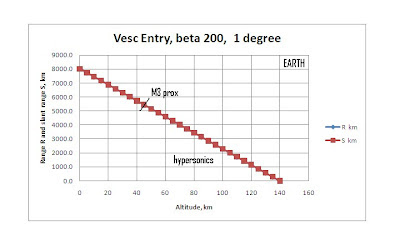Figure 4 – Earth Entry Range and Slant Range vs. AltitudeFigure 5 – Earth Entry Deceleration Gee Profile vs. Slant RangeFigure 6 – Earth Entry Heating Traces vs. Slant Range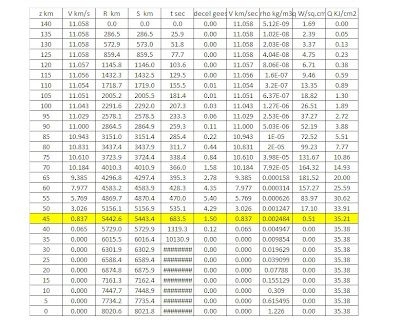Figure 7 – Image of Earth Entry Spreadsheet Data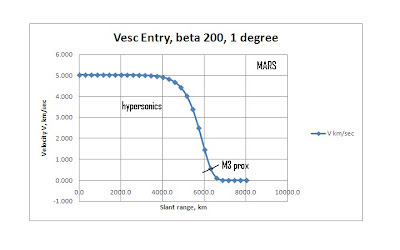Figure 8 – Mars Entry Velocity Profile vs. Slant Range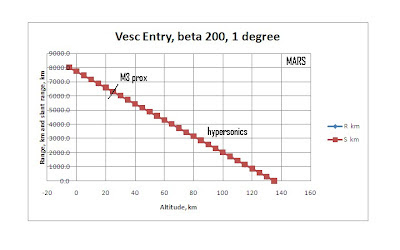Figure 9 – Mars Entry Range and Slant Range vs. AltitudeFigure 10 – Mars Entry Deceleration Gee Profile vs. Slant Range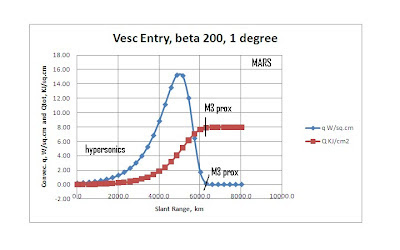Figure 11 – Mars Entry Heating Traces vs. Slant Range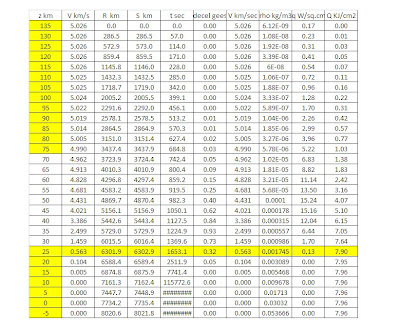Figure 12 – Image of Mars Entry Spreadsheet Data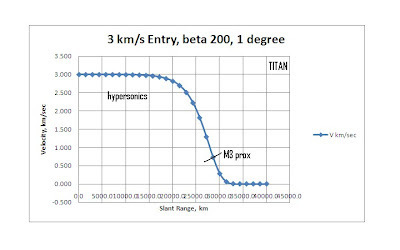Figure 13 – Titan Entry Velocity Profile vs. Slant RangeFigure 14 – Titan Entry Range and Slant Range vs. Altitude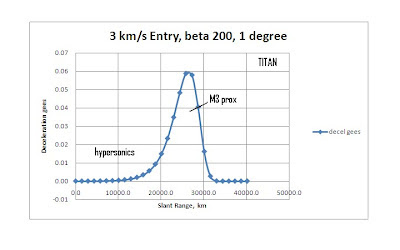Figure 15 – Titan Entry Deceleration Gee Profile vs. Slant Range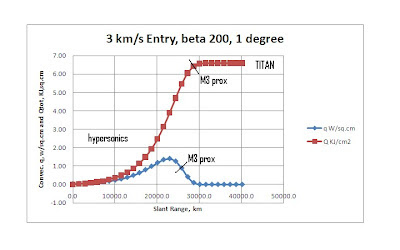Figure 16 – Titan Entry Heating Traces vs. Slant Range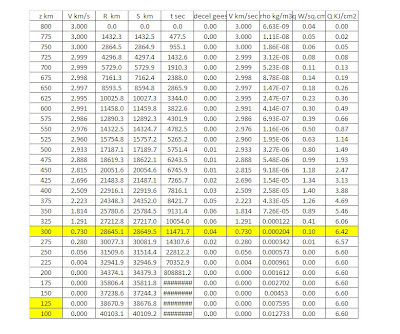Figure 17 – Image of Titan Entry Spreadsheet Data